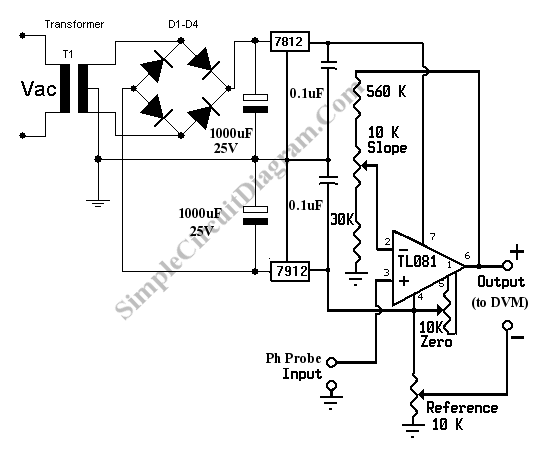# Simple pH Meter Circuit, A Low Cost Adapter for Your Digital Voltmeter

What is A pH Meter?

A pH meter is actually a precise voltmeter  that measures the generated voltage of a pH electrodes. The requirement of such meter is high input impedance and has the gain of  voltage-pH conversion. The standard pH probe generate voltage about 59 mV per pH,  so all we need is a pre-amplifier with high impedance input and with gain = 16.7 to give 1 Volt per pH. Here is the schematic diagram of the pH meter circuit:pH Meter Circuit

This pH meter circuit use a high input impedance high gain op-amp TL081. The transformer voltage is center tapped 15V  (15V-0V-15V). Multi-turn variable resistor is recommended for the zero, slope, and reference variable resistors. Current consumption of this pH meter circuit should be very small, so the 7812 and 7912 regulator IC won’t need any heat sink.

pH Meter Calibration

To calibrate our pH meter circuit,  follow these steps:

• Null the amplifier by shorting the pH probe input to ground. Measure the voltage between output + and ground. Adjust the zero potentiometer until the voltmeter reading show zero volt (or close to zero).
• Now connect pH probe  input to a 413 mV voltage source. You can use a potentiometer to make a  simple 413 mV voltage source. After connecting the input to 413 mV source, then measure the  + output  and ground using the digital voltmeter  and you should read 7.00 volt. If not then adjust the slope potentiometer until you get the closest reading to 7.00 volts.
• Short the pH probe input to ground. Connect the digital voltmeter voltmeter between – output and + output. Now adjust reference potentiometer until the voltmeter show 7.00 volts. This corresponds to the neutral pH of 7.
• Connect the pH probe input to a pH electrodes (pH probe/sensor), now the pH meter is ready to use. The voltmeter reading in volts would be direct conversion of pH. pH value of 7 would be read as 7 volts, pH 3 as 3 volts, etc.

With low cost digital voltmeter (DVM), we can easily obtain 0.05 pH accuracy if  we follow the calibration method as described above.